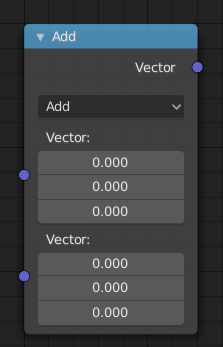# Vector Math Node¶Vector Math Node.

The Vector Math node performs the selected math operation on the input vectors.

## Inputs¶

The inputs of the node are dynamic. Some inputs are only available in certain operations. For instance, the Scale input is only available in the Scale operator.

Vector

Input vector $$A = \begin{pmatrix} A_x \\ A_y \\ A_z \end{pmatrix}$$.

Vector

Input vector $$B = \begin{pmatrix} B_x \\ B_y \\ B_z \end{pmatrix}$$.

Scale

Input Scale $$s$$.

## Properties¶

Operation

The vector math operator to be applied on the input vectors.

The sum of A and B. $$\begin{pmatrix} A_x + B_x \\ A_y + B_y \\ A_z + B_z \end{pmatrix}$$

Subtract

The difference between A and B. $$\begin{pmatrix} A_x - B_x \\ A_y - B_y \\ A_z - B_z \end{pmatrix}$$

Multiply

The entrywise product of A and B. $$\begin{pmatrix} A_x \cdot B_x \\ A_y \cdot B_y \\ A_z \cdot B_z \end{pmatrix}$$

Divide

The entrywise division of A by B. Division by zero results in zero. $$\begin{pmatrix} A_x / B_x \\ A_y / B_y \\ A_z / B_z \end{pmatrix}$$

Cross Product

The cross product of A and B. $$\begin{pmatrix} A_y \cdot B_z - A_z \cdot B_y \\ A_z \cdot B_x - A_x \cdot B_z \\ A_x \cdot B_y - A_y \cdot B_x \end{pmatrix}$$

Project

The projection of A onto B.

Reflect

The reflection of A around the normal B. B need not be normalized.

Dot Product

The dot product of A and B. $$A_x \cdot B_x + A_y \cdot B_y + A_z \cdot B_z$$

Distance

The distance between A and B.

Length

The length of A. $$\sqrt{A_x^2 + A_y^2 + A_z^2}$$

Scale

The result of multiplying A by the scalar input Scale. $$\begin{pmatrix} s \cdot A_x \\ s \cdot A_y \\ s \cdot A_z \end{pmatrix}$$

Normalize

The result of normalizing A. The result vector points to the same direction as A and has a length of 1. If A is (0, 0, 0), the result is (0, 0, 0) as well.

Wrap

Wrap.

Snap

The result of rounding A to the largest integer multiple of B less than or equal A.

Floor

The entrywise floor of A.

Ceil

The entrywise ceiling of A.

Modulo

The entrywise modulo of A by B.

Fraction

The fractional part of A.

Absolute

The entrywise absolute value of A.

Minimum

The entrywise minimum from A and B.

Maximum

The entrywise maximum from A and B.

Sine

The entrywise Sine of A.

Cosine

The entrywise Cosine of A.

Tangent

The entrywise Tangent of A.

## Outputs¶

The output of the node is dynamic. It is either a vector or a scalar depending on the operator. For instance, the Length operator has a scalar output while the Add operator has a vector output.

Vector

Output vector.

Value

Output value.